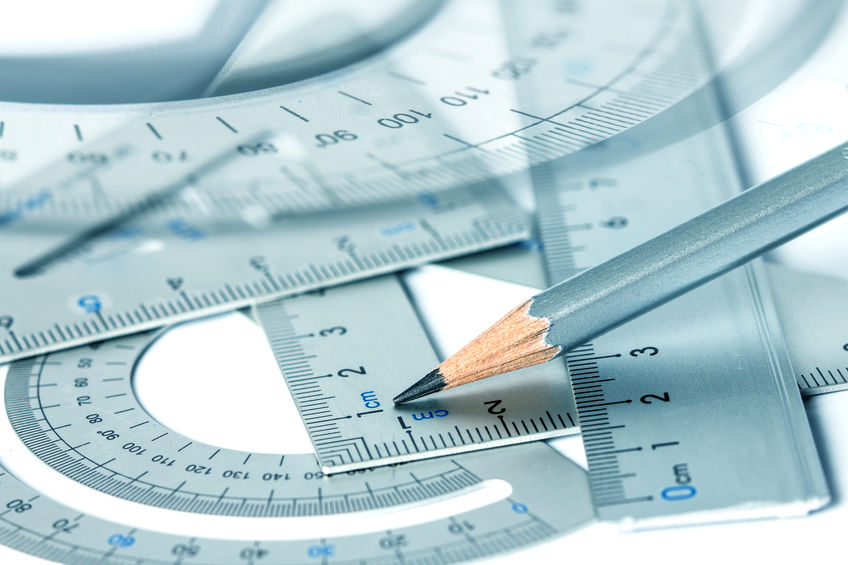# The Blog of Mathedu Academy

### A content for the curious of the mathematics in the real world… and beyond# Distance Units Conversion and Orders of Magnitude

The International System distance measurement unit is the meter. But in everyday life, we use many other distance measurement units. How is it possible to express any distance, whatever its order of magnitude or its usage measurement unit, in meters?

For the so called "metric system", we use the multiplication or division by a power of $$10$$, say $$10^k$$, that is $$10$$ multiplied by itself a given number $$k$$ of times.

For instance, as $$1 \; cm$$ = $$\frac{1}{100} \; m$$, we have to
divide a distance in centimetres by $$100 = 10^2$$, to obtain the same distance in meters. For instance,$$25 \; cm = 0.25 \; m$$.

For a distance between cities, we may use kilometres or miles. In the metric system, it is kilometers ( $$km$$ ), and $$1 \; km = 1,000 \; m$$. So we have to
multiply a distance in kilometres by $$1,000 = 10^3$$, to obtain the same distance in meters. For instance: $$150 \; km = 150,000 \; m$$.

For cellulars, scientists use the micron as a standard unit. Is is one thousands of millimetres, or one millionth of meters. Thus we have to divide a size in microns by $$1,000,000 = 10^6$$, to obtain the same distance in meters.

But we have a problem with such a number, because $$3$$ microns is equal to $$0.000,003 \; m$$, and this is not easy to read. Thus we use the scientific notation, that is on your calculator $$3E-6$$ for $$3 \times 10^{-6}$$, the inverse of $$10^6$$, one million.

The "big" numbers are also denoted with the scientific notation. For instance, the speed light is about $$3E{+8} \; m/s$$ ( $$3 \times 10^8$$ meters per second), the international speed unit. If we divide it by $$1,000$$,
that is $$10^3$$, the power of $$10$$ become $$8-3=5$$, so we see that it is about $$3E{+5} = 300,000 \; km/s$$, which we already know.

The order of magnitude of a number is roughly the power of $$10$$ in its scientific notation, the bigger it is, the much bigger the number is, and the most negative it is, the tiniest the number is (closer and closer to $$0$$ ).

Update 02/04/2020: A new post is dedicated to scientific notation
here
Back